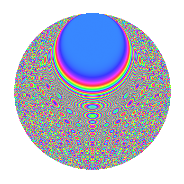# Properties

 Label 1575.2.cfLevel 1575 Weight 2 Character orbit cf Rep. character $$\chi_{1575}(32,\cdot)$$ Character field $$\Q(\zeta_{12})$$ Dimension 560 Sturm bound 480

# Related objects

## Defining parameters

 Level: $$N$$ = $$1575 = 3^{2} \cdot 5^{2} \cdot 7$$ Weight: $$k$$ = $$2$$ Character orbit: $$[\chi]$$ = 1575.cf (of order $$12$$ and degree $$4$$) Character conductor: $$\operatorname{cond}(\chi)$$ = $$315$$ Character field: $$\Q(\zeta_{12})$$ Sturm bound: $$480$$

## Dimensions

The following table gives the dimensions of various subspaces of $$M_{2}(1575, [\chi])$$.

Total New Old
Modular forms 1008 592 416
Cusp forms 912 560 352
Eisenstein series 96 32 64

## Trace form

 $$560q$$ $$\mathstrut +\mathstrut 6q^{2}$$ $$\mathstrut +\mathstrut 2q^{3}$$ $$\mathstrut +\mathstrut 2q^{7}$$ $$\mathstrut +\mathstrut O(q^{10})$$ $$560q$$ $$\mathstrut +\mathstrut 6q^{2}$$ $$\mathstrut +\mathstrut 2q^{3}$$ $$\mathstrut +\mathstrut 2q^{7}$$ $$\mathstrut +\mathstrut 22q^{12}$$ $$\mathstrut +\mathstrut 4q^{13}$$ $$\mathstrut +\mathstrut 260q^{16}$$ $$\mathstrut +\mathstrut 18q^{17}$$ $$\mathstrut +\mathstrut 10q^{18}$$ $$\mathstrut -\mathstrut 64q^{21}$$ $$\mathstrut -\mathstrut 4q^{22}$$ $$\mathstrut +\mathstrut 32q^{27}$$ $$\mathstrut +\mathstrut 4q^{28}$$ $$\mathstrut +\mathstrut 4q^{31}$$ $$\mathstrut +\mathstrut 90q^{32}$$ $$\mathstrut -\mathstrut 32q^{33}$$ $$\mathstrut -\mathstrut 16q^{36}$$ $$\mathstrut +\mathstrut 4q^{37}$$ $$\mathstrut -\mathstrut 14q^{42}$$ $$\mathstrut +\mathstrut 4q^{43}$$ $$\mathstrut -\mathstrut 32q^{46}$$ $$\mathstrut +\mathstrut 6q^{47}$$ $$\mathstrut -\mathstrut 38q^{48}$$ $$\mathstrut -\mathstrut 52q^{51}$$ $$\mathstrut +\mathstrut 52q^{52}$$ $$\mathstrut +\mathstrut 156q^{56}$$ $$\mathstrut -\mathstrut 32q^{57}$$ $$\mathstrut +\mathstrut 12q^{58}$$ $$\mathstrut +\mathstrut 28q^{61}$$ $$\mathstrut -\mathstrut 14q^{63}$$ $$\mathstrut +\mathstrut 244q^{66}$$ $$\mathstrut -\mathstrut 2q^{67}$$ $$\mathstrut +\mathstrut 46q^{72}$$ $$\mathstrut +\mathstrut 4q^{73}$$ $$\mathstrut -\mathstrut 24q^{76}$$ $$\mathstrut -\mathstrut 42q^{77}$$ $$\mathstrut -\mathstrut 54q^{78}$$ $$\mathstrut -\mathstrut 52q^{81}$$ $$\mathstrut +\mathstrut 8q^{82}$$ $$\mathstrut +\mathstrut 12q^{83}$$ $$\mathstrut +\mathstrut 28q^{87}$$ $$\mathstrut -\mathstrut 12q^{88}$$ $$\mathstrut -\mathstrut 16q^{91}$$ $$\mathstrut -\mathstrut 72q^{92}$$ $$\mathstrut -\mathstrut 4q^{93}$$ $$\mathstrut +\mathstrut 36q^{96}$$ $$\mathstrut +\mathstrut 4q^{97}$$ $$\mathstrut -\mathstrut 12q^{98}$$ $$\mathstrut +\mathstrut O(q^{100})$$

## Decomposition of $$S_{2}^{\mathrm{new}}(1575, [\chi])$$ into irreducible Hecke orbits

The newforms in this space have not yet been added to the LMFDB.

## Decomposition of $$S_{2}^{\mathrm{old}}(1575, [\chi])$$ into lower level spaces

$$S_{2}^{\mathrm{old}}(1575, [\chi]) \cong$$ $$S_{2}^{\mathrm{new}}(315, [\chi])$$$$^{\oplus 2}$$